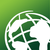# Widget request/info: calculate density by drawing a polygon and dividing the sum of points by the polygon size.

212
0
12-09-2022 10:42 AMby
New Contributor

I’m looking for a widget, or if none exists requesting one, where you can draw a polygon to select points or polygons. A chosen attribute or count of the selected points/polygons is summed, then that sum is divided by the size of the drawn polygon and the result is displayed along with the sum itself and the sum of the polygon. The only thing a user should have to do is draw a polygon, then the result should display by itself. user should be able to choose which layer the polygon should select and which attribute in that layer to sum. The user should also be able to select which measurement be used as the size of the polygon, e.g sq m, sq feet etc and the format for the results, e.g. as a percentage, per sq m, sq ft, sq km, sq mi etc. An added bonus would be to also have a feature where you could also use a feature in a polygon layer as the selection layer instead of drawing.

The widget could be used as sort of a floor area ratio calculator or density calculator.

For example as a floor area ratio calculator, if there’s a building points layer with a floor area (total floor area for each floor in the building) attribute in sq m. Then I’d want to draw a polygon, then the widget sums the floor area for the buildings, then divides that by the size (in sq m) of the polygon, and displays the result as a percentage, with the floor area sum and polygon size underneath or to the side in a smaller font.

Does a widget like this, or widgets that can be used together, exist? If not, I’d like to put in a request for a widget like this. If  you can also select the size units it could be used as a density calculator for a lot of useful things. Like population, workplaces, schools, grocery stores, sport facilities, floor area ratio and a range of other urban planning analyses and in addition various environmental densities and probably lots of other fields.

0 Replies